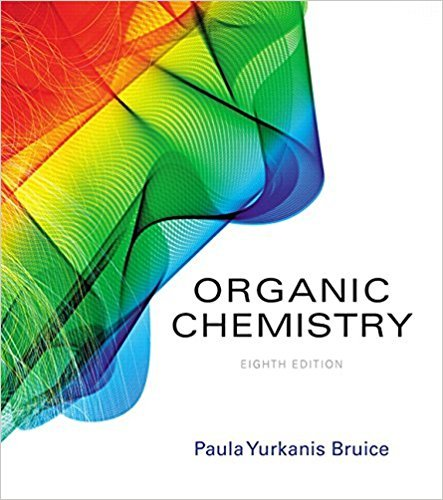×
×

# Solutions for Chapter 26: The Chemistry of the Nucleic Acids## Full solutions for Organic Chemistry | 8th Edition

ISBN: 9780134042282Solutions for Chapter 26: The Chemistry of the Nucleic Acids

Solutions for Chapter 26
4 5 0 384 Reviews
22
2
##### ISBN: 9780134042282

This expansive textbook survival guide covers the following chapters and their solutions. Chapter 26: The Chemistry of the Nucleic Acids includes 39 full step-by-step solutions. Organic Chemistry was written by and is associated to the ISBN: 9780134042282. Since 39 problems in chapter 26: The Chemistry of the Nucleic Acids have been answered, more than 36254 students have viewed full step-by-step solutions from this chapter. This textbook survival guide was created for the textbook: Organic Chemistry, edition: 8.

Key Chemistry Terms and definitions covered in this textbook
• acidic anhydride (acidic oxide)

An oxide that forms an acid when added to water; soluble nonmetal oxides are acidic anhydrides. (Section 22.5)

• atomic weight

The average mass of the atoms of an element in atomic mass units (amu); it is numerically equal to the mass in grams of one mole of the element. (Section 2.4)

A statement that equal volumes of gases at the same temperature and pressure contain equal numbers of molecules. (Section 10.3)

• azide synthesis

A method for preparing primary amines that avoids the formation of secondary and tertiary amines.

• Bicycloalkane

An alkane containing two rings that share two carbons

In 13C NMR spectroscopy, a technique in which all 13C!1H splitting is suppressed with the use of two rf transmitters.

• chemically equivalent

In NMR spectroscopy, protons (or carbon atoms) that occupy identical electronic environments and produce only one signal.

• chemistry

The scientific discipline that studies the composition, properties, and transformations of matter. (Chapter 1: Introduction)

• Claisen rearrangement

A [3,3] sigmatropic rearrangement that is observed for allylic vinylic ethers.

• cycloalkane

An alkane whose structure contains a ring.

• diatomic molecule.

A molecule that consists of two atoms. (2.5)

• dipole

A molecule with one end having a partial negative charge and the other end having a partial positive charge; a polar molecule. (Section 8.4)

• Disaccharide

A carbohydrate containing two monosaccharide units joined by a glycosidic bond.

• Levorotatory

Refers to a substance that rotates the plane of polarized light to the left.

• microstate

The state of a system at a particular instant; one of many possible energetically equivalent ways to arrange the components of a system to achieve a particular state. (Section 19.3)

• Nucleic acid

A biopolymer containing three types of monomer units: heterocyclic aromatic amine bases derived from purine and pyrimidine, the monosaccharides d-ribose or 2-deoxy-d-ribose, and phosphoric acid

• nucleic acids

Polymers of high molecular weight that carry genetic information and control protein synthesis. (Section 24.10)

• Nucleotide

A nucleoside in which a molecule of phosphoric acid is esterifi ed with an !OH of the monosaccharide, most commonly either the 39!OH or the 59!OH.

• second-order reaction

A reaction in which the overall reaction order (the sum of the concentration- term exponents) in the rate law is 2. (Section 14.4)

• Ylide

A neutral molecule with positive and negative charges on adjacent atoms

×